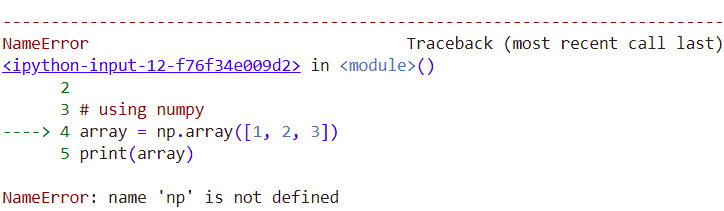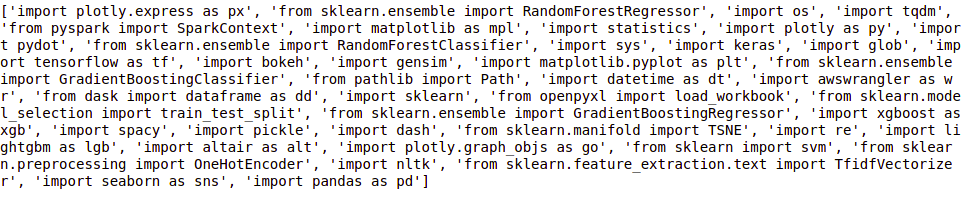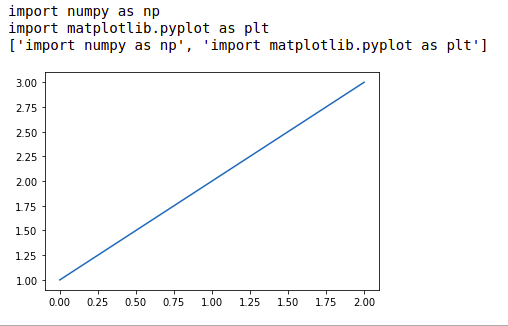Open In App

# Lazy import in Python

In this article, we will learn how to do Lazy import in Python. For this, let’s first understand the lazy import.

## What is Lazy Import?

It is a feature of the Pyforest module that allows the user to perform the task without adding libraries to the code snippet as the task of this function is to add those libraries themselves into the code snippet. It was designed for the users who are tired of writing those import statements in the program. It is very useful for the developers who need to write long lengthy codes it gives an edge to them.

## Why we need Lazy Import?

As a Python user, we often face the problem of importing multiple modules such as NumPy, pandas, sklearn, nltk, os, sys, pickle, and many others in every program every time which is actually annoying and irritating as we are doing some work which is no benefit, rather is a wastage of time and if any time we forgot to import any module in the program then the program gives an error, and we don’t want our program to crash just because of the modules.

So Pyforest has made a solution for this problem by creating an in-built function that has the details of almost 99% of the popular modules of Python which identifies the imports we are using and automatically adds the module for the program so that we don’t need to do that again and again. This way it is easier and faster because we can use the pre-installed modules without importing them into different programs, and it also doesn’t add all the modules it checks the program and only the used modules will be added, so there is no such a disadvantage at the ease that we do not need to add those modules, again and again, our work gets done by adding just a single module.

Example:

Let’s write some code of lines, and we don’t import the required module. This is our simple and continuous habit.

## Python3

 `# forget to import numpy`` ` `# using numpy``array ``=` `np.array([``1``, ``2``, ``3``])``print``(array)`

If we run the above piece of code we will get the following error :Now, we get the error as the module is not imported. In this similar way, we forget to import the required modules in big lines of codes. To import we have to write importing statements for each and every module used in our code. So, do this in one line we use lazy_import from pyforest module.

### Installation of pyforest Module

For the installation process to begin we need to have python installed on our device, then we need to open the command prompt and write the following command in the prompt to install the ‘pyforest’ module which gives us the functionality of the lazy_module().

```Installation:
pip install pyforest## Importing and Lazy_import

To see the import function in pyforest module we can print the lazy_modules() function:

## Python3

 `import` `pyforest`` ` ` ` `print``(lazy_imports())`

Output:So we can see in the above output what all modules are existing in the pyforest library which is available for use without importing multiple times.

This was just one line but if we had to import a lot of modules we will have to focus more on importing modules rather than writing the code. So here Pyforest solves the problem. Just add one module ‘import pyforest’ and we are ready to go.

Example 1:

## Python3

 `# forget to import numpy``# nut importing pyforest``import` `pyforest`` ` `# using numpy``array ``=` `np.array([``1``, ``2``, ``3``])``print``(array)`` ` `print``(active_imports())`

Output:

```[1 2 3]
import numpy as np
['import numpy as np']```

Example 2:

## Python3

 `# forget to import numpy, matplotlib``# but importing pyforest``import` `pyforest`` ` `# using numpy``array ``=` `np.array([``1``, ``2``, ``3``])`` ` `# using matplotlib.pyplot``plt.plot(array)`` ` `print``(active_imports())`

Output:Lazy import is a very useful feature of the Pyforest library as this feature automatically imports the library for us, if we don’t use the library it won’t be added. This feature is very useful to those who don’t want to write the import statements again and again in their code. The main benefit of this library is that it contains most of the libraries, and we can use the library as we like anytime without importing that library as the lazy import feature will do it for us.